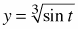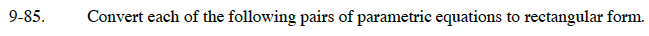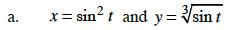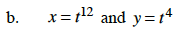### Home > CALC > Chapter 9 > Lesson 9.3.1 > Problem9-85

9-85.
1. Convert each of the following pairs of parametric equations to rectangular form. Homework Help ✎

1. x = sin2 t and2. x = t12 and y = t4$(\sin^{1/3}(t))^6=\sin^2(t)$$(t^4)^3=t^{12}$